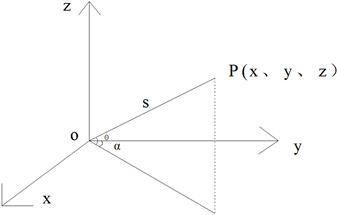# 三维扫描仪在河道勘测中的对比分析Contrastive Analysis of Three-Dimensional Scanner in River Survey

DOI: 10.12677/JWRR.2019.84046, PDF, HTML, XML, 下载: 439  浏览: 783

Abstract: Ground 3D laser scanning was used to collect 3D coordinate data of the reflectorperform point cloud data processing and mathematical modeling. Two kinds of three-dimensional laser scanning instruments, namely Haida HS1200 and TrimbleSX10, were selected for experimental research. The error statistical analysis showed that the former had a large amount of scanning points and could be upgraded to shipborne mode. CAD drawing was fast; TimbleSX10 scanning point data volume was small, and its operation was concise; but CAD mapping needed to be improved. The two have great advantages in monitoring hillside cliffs and bank collapse, but non-sand beaches, bare land and other areas need further attention and experimentation.

1. 引言

2. 系统概况及扫测原理

2.1. 系统概况

2.2. 扫测原理Figure 1. 3d laser scanner internal coordinate system

$\left\{\begin{array}{l}X=S\mathrm{cos}\theta \mathrm{cos}\alpha \\ Y=S\mathrm{cos}\theta \mathrm{cos}\alpha \\ Z=S\mathrm{sin}\theta \end{array}$ (1)

3. 数据采集

3.1. 中海达HS1200采集

1) 控制测量

2) 点云数据采集

① 扫描的有效距离约500 m。

② 每一站仅扫描耗时约20分钟，总计耗时约2小时。

③ 布设控制点8个。

④ 总计扫描面积约0.3 km2

3.2. TrimbleSX10采集

1) 控制测量

2) 点云数据采集

TrimbleSX10共施测4站。主要有以下情况：

① 扫描的距离约500 m。

② 每一站仅扫描耗时约20分钟，总计耗时约3小时。

③ 布设控制点4个。

④ 总计扫描面积约0.1 km2，地形区域复杂。

4. 数据处理与统计

4.1. 数据处理

4.1.1. 点云数据处理

4.1.2. 数学建模与成图

1) 点云数据的建模

2) 点云重采样

3) 点云的坐标转换

4) 地形成图

4.2. 数据统计与分析

4.2.1. 数据统计Figure 2. Map of typical waterfront area

${M}_{x,y}=±\sqrt{\frac{\underset{i=1}{\overset{n}{\sum }}\left({\left({{X}^{\prime }}_{i}-{X}_{i}\right)}^{2}+{\left({{Y}^{\prime }}_{i}-{Y}_{i}\right)}^{2}\right)}{n-1}}$ (2)

${M}_{h}=±\sqrt{\frac{\underset{i=1}{\overset{n}{\sum }}{\left({{H}^{\prime }}_{i}-{H}_{i}\right)}^{2}}{n-1}}$ (3)

1) 无遮挡区域地面测点较差统计

① 平面较差统计Table 1. Characteristics of different ways of measuring point difference statistics

② 高程互差统计如表2Table 2. Different ways of measuring height feature points difference statistic values

③ 计算中误差

${M}_{x,y}=0.054\text{\hspace{0.17em}}\text{m}$

${M}_{h}=0.025\text{\hspace{0.17em}}\text{m}$

2) 遮挡植被区域地形点高程较差对比

4.2.2. 分析结论

1) 裸露地表进行三维激光扫描的平面及高程精度较高，满足大比例尺测图的精度。

2) 含有植被的区域误差与植被高度有关，特别是密实的植被区域，不能采用此方法作业。

5. 误差分析

1) 植被对高程的影响

2) 点云数据拼接影响

3) 其他影响因素

6. 结论